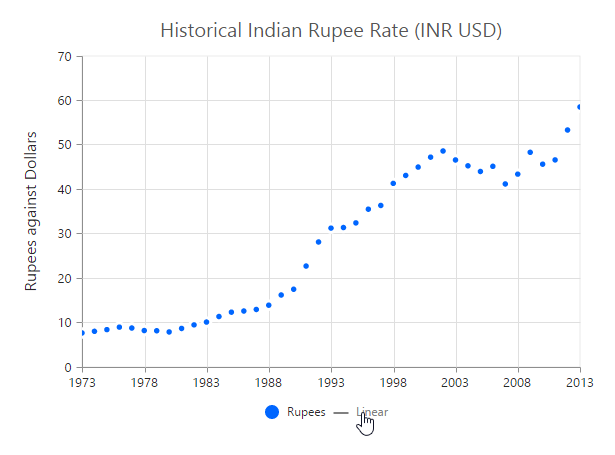# Trendlines support in Angular Chart

28 Oct 20227 minutes to read

EjChart can generate Trendlines for Cartesian type series (Line, Column, Scatter, Area, Candle, HiLo etc.) except bar type series. You can add more than one trendline object to the `trendlines` option.

• TS
• ``````this.ChartTrendlines= [{
//Enable Trendline to chart series
visibility: "visible", type: "linear"
}];``````
• HTML
• ``````<ej-chart id="chartcontainer">
<e-seriescollection>
<e-series [trendlines]="ChartTrendlines">
</e-series>
</e-seriescollection>
</ej-chart>``````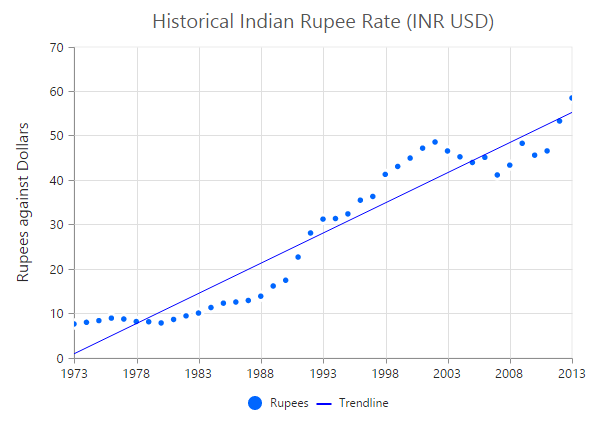## Customize the trendline styles

A trendline can be customized by using the properties such as `fill`, `width`, dashArray and opacity. The default type of trendline is “linear”.

• TS
• ``````this.ChartTrendlines = [{
//...
//Customize the Trendline styles
fill: '#99CCFF', width: 3, opacity: 1, dashArray: '2,3'
}];``````
• HTML
• ``````<ej-chart id="chartcontainer">
<e-seriescollection>
<e-series [trendlines]="ChartTrendlines">
</e-series>
</e-seriescollection>
</ej-chart>``````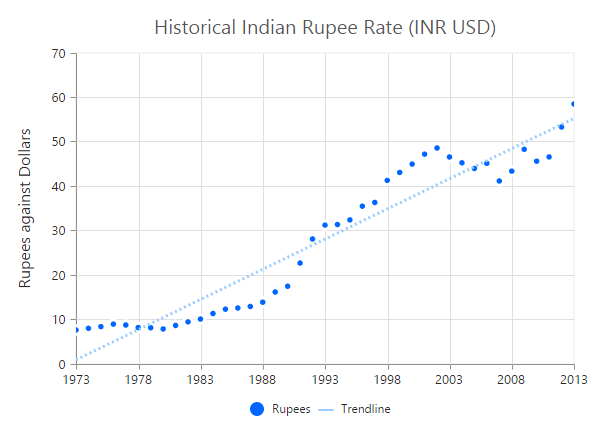## Types of Trendline

EjChart supports the following type of Trendlines.

• Linear
• Exponential
• Logarithmic
• Power
• Polynomial
• MovingAverage

### Linear

To render Linear Trendline, you have to set the `type` as “linear”.

• TS
• ``````this.ChartTrendlines = [{
//Change Trendline type
type: "linear"
}];``````
• HTML
• ``````<ej-chart id="chartcontainer">
<e-seriescollection>
<e-series [trendlines]="ChartTrendlines">
</e-series>
</e-seriescollection>
</ej-chart>``````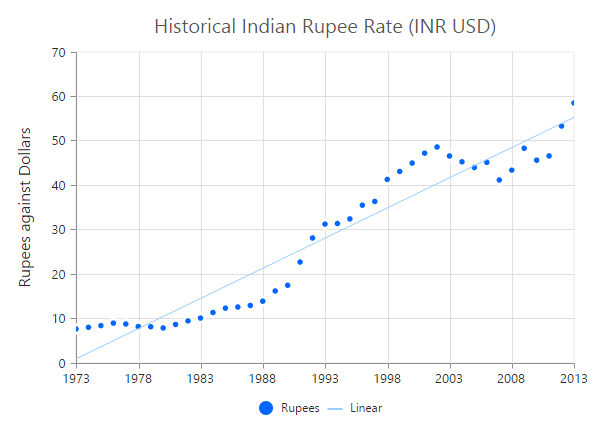### Exponential

Exponential Trendline can be rendered by setting the `type` as “exponential”.

• TS
• ``````this.ChartTrendlines = [{
//Change Trendline type
type: "exponential"
}];``````
• HTML
• ``````<ej-chart id="chartcontainer">
<e-seriescollection>
<e-series [trendlines]="ChartTrendlines">
</e-series>
</e-seriescollection>
</ej-chart>``````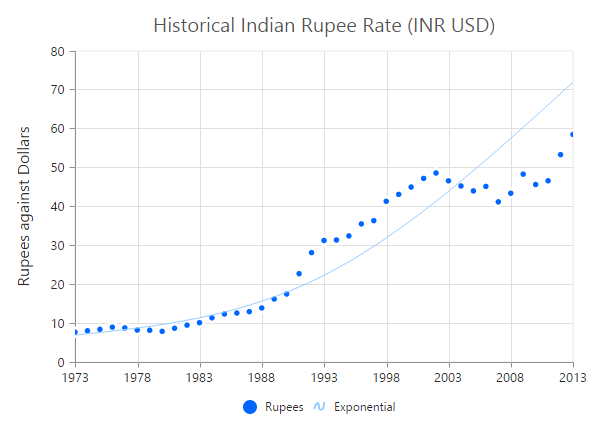### Logarithmic

Logarithmic Trendline can be rendered by setting the `type` as “Logarithmic”.

• TS
• ``````this.ChartTrendlines = [{
//Change Trendline type
type: "logarithmic"
}];``````
• HTML
• ``````<ej-chart id="chartcontainer">
<e-seriescollection>
<e-series [trendlines]="ChartTrendlines">
</e-series>
</e-seriescollection>
</ej-chart>``````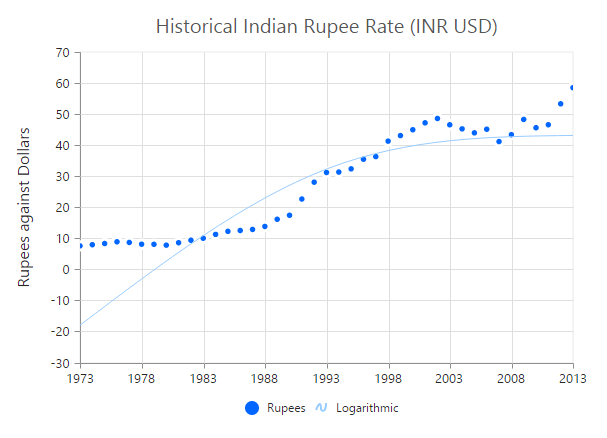### Power

Power Trendline can be rendered by setting the `type` of the trendline as “power”.

• TS
• ``````this.ChartTrendlines = [{
//Change Trendline type
type: "power"
}];``````
• HTML
• ``````<ej-chart id="chartcontainer">
<e-seriescollection>
<e-series [trendlines]="ChartTrendlines">
</e-series>
</e-seriescollection>
</ej-chart>``````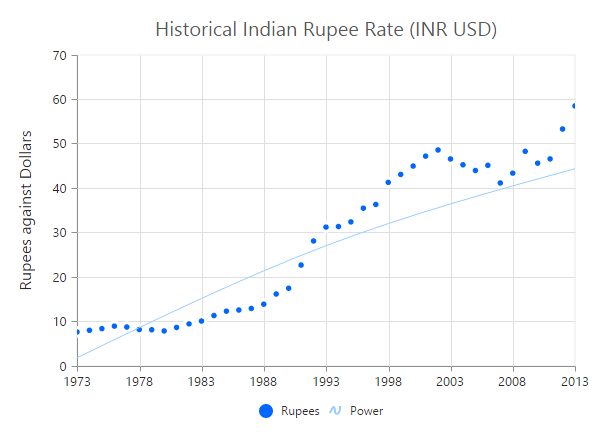### Polynomial

Polynomial Trendline can be rendered by setting the trendline `type` as “polynomial”. You can change the polynomial order by using the polynomialOrder of the trendlines. It ranges from 2 to 6.

• TS
• ``````this.ChartTrendlines = [{
//Change Trendline type
type: "polynomial"
}];``````
• HTML
• ``````<ej-chart id="chartcontainer">
<e-seriescollection>
<e-series [trendlines]="ChartTrendlines">
</e-series>
</e-seriescollection>
</ej-chart>``````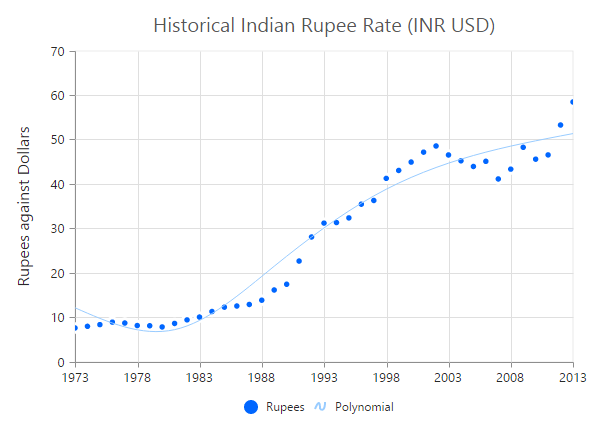### MovingAverage

MovingAverage Trendline can be rendered by setting the `type` of the trendline as “movingAverage”.

• TS
• ``````this.ChartTrendlines = [{
//Change Trendline type and set period for moving average
type: "movingAverage", period: 3
}];``````
• HTML
• ``````<ej-chart id="chartcontainer">
<e-seriescollection>
<e-series [trendlines]="ChartTrendlines">
</e-series>
</e-seriescollection>
</ej-chart>``````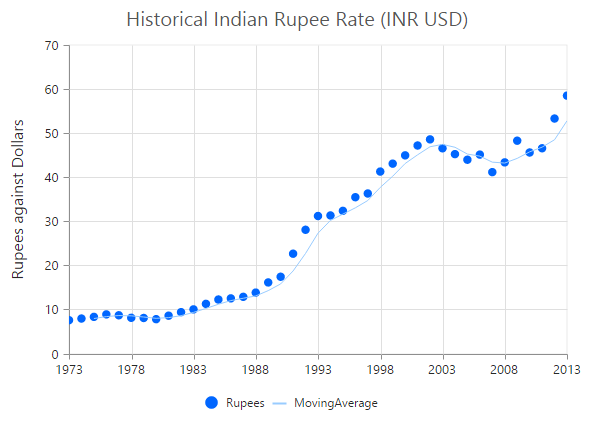## Forecasting

Trendline forecasting is the prediction of future/past situations. Forecasting can be classified into two types as follows.

• Forward Forecasting
• Backward Forecasting

### Forward Forecasting

The value set for `forwardForecast` is used to determine the distance moving towards the future trend.

• TS
• ``````this.ChartTrendlines = [{
//...
//Set forward forecasting value
forwardForecast: 5
}];``````
• HTML
• ``````<ej-chart id="chartcontainer">
<e-seriescollection>
<e-series [trendlines]="ChartTrendlines">
</e-series>
</e-seriescollection>
</ej-chart>``````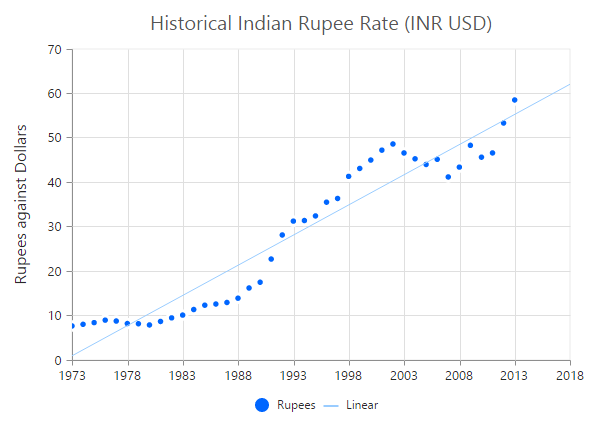### Backward Forecasting

The value set for the `backwardForecast` is used to determine the past trends.

• TS
• ``````this.ChartTrendlines = [{
//...
//Set forward forecasting value
backwardForecast: 5
}];``````
• HTML
• ``````<ej-chart id="chartcontainer">
<e-seriescollection>
<e-series [trendlines]="ChartTrendlines">
</e-series>
</e-seriescollection>
</ej-chart>``````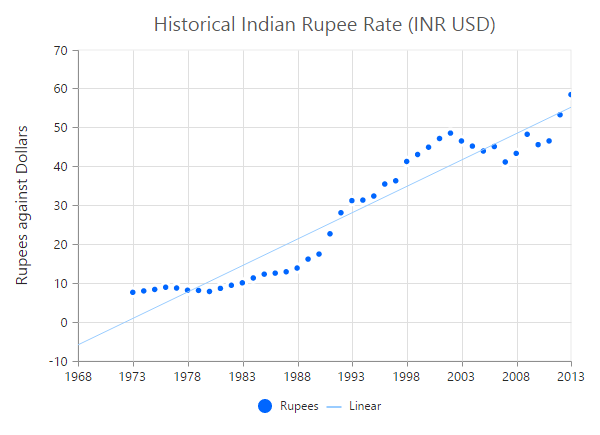## Trendlines Legend

To display the legend item for trendline, use the `name` property. You can interact with the trendline legends similar to the series legends (show/hide trendlines on legend click).

• TS
• ``````this.ChartTrendlines = [{
//...
//Set Trendline name to display in the legend
name: 'Linear'
}];``````
• HTML
• ``````<ej-chart id="chartcontainer">
<e-seriescollection>
<e-series [trendlines]="ChartTrendlines">
</e-series>
</e-seriescollection>
</ej-chart>``````